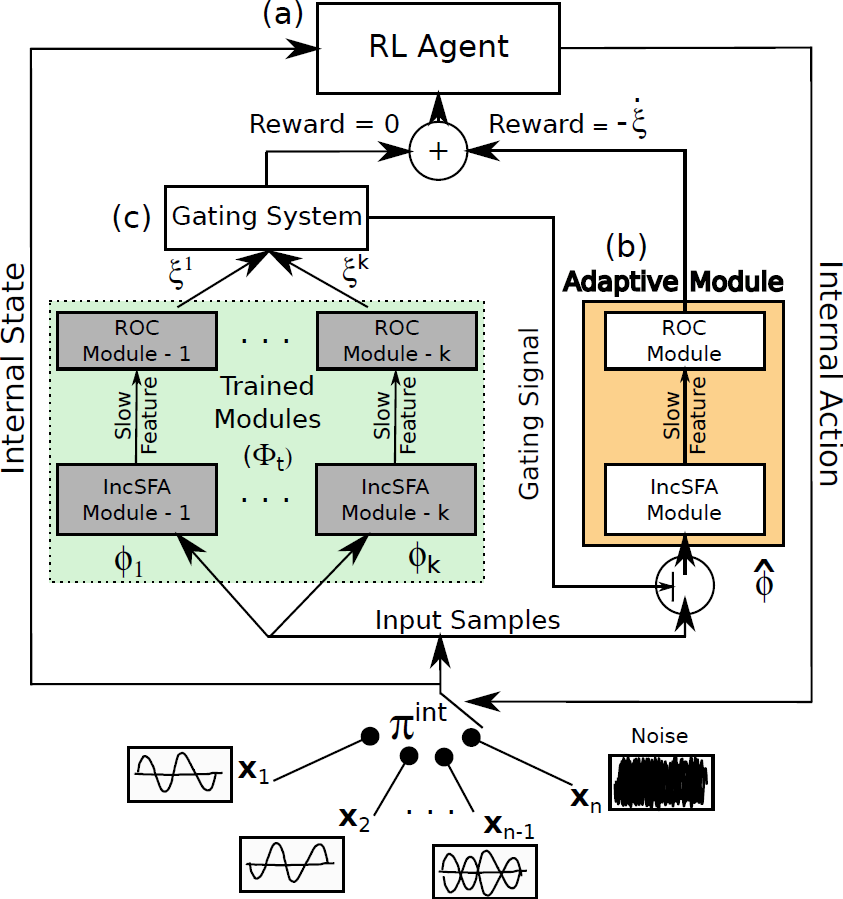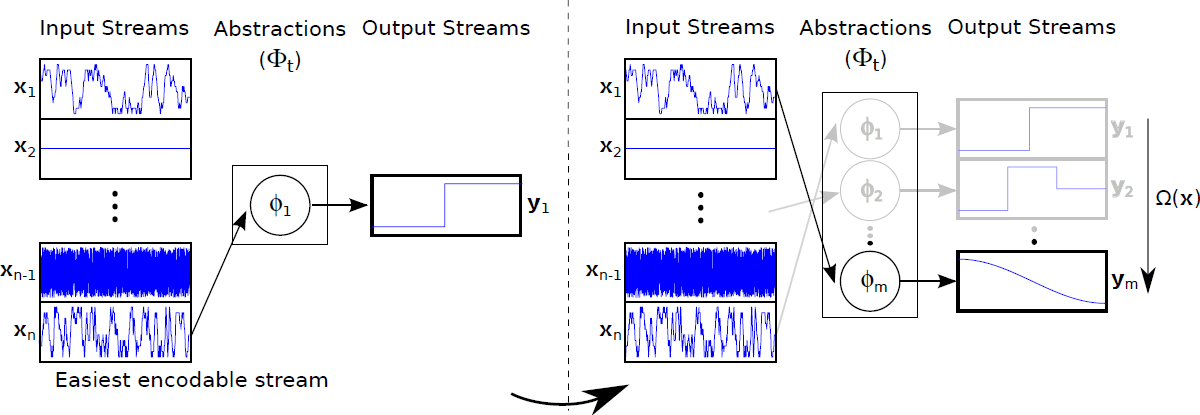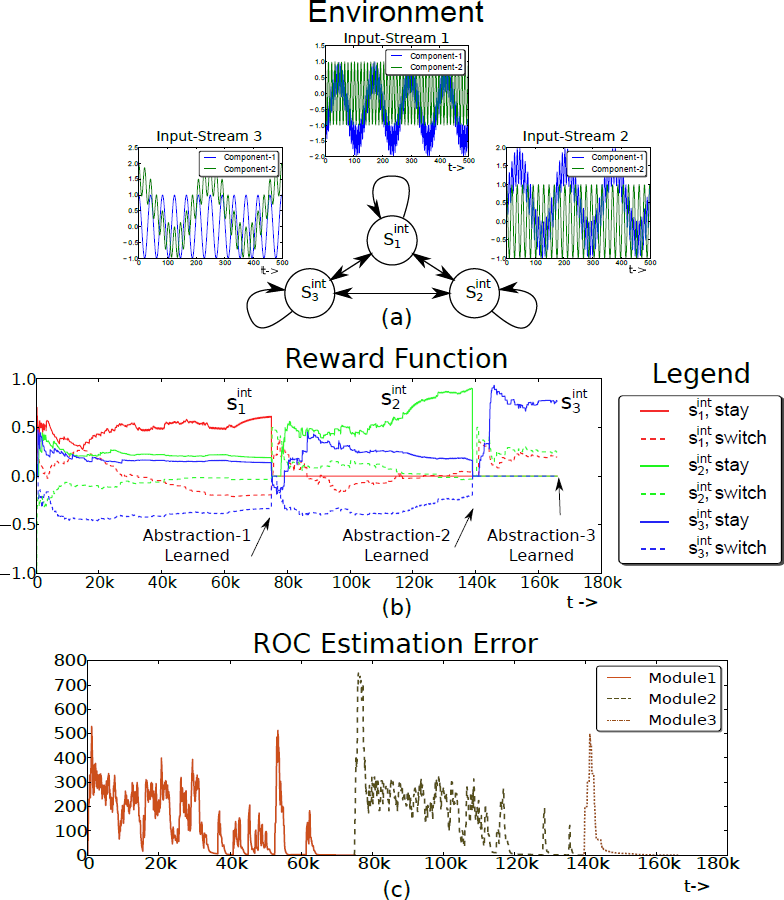<- Home

## OverviewFigure 4: Architecture of Curious Dr.\ MISFA includes (a) a reinforcement learning agent that generates an observation stream selection policy based on the intrinsic rewards, (b) an adaptive Incremental SFA coupled with Robust Online Clustering module that updates an abstraction based on the incoming observations, and (c) a gating system that prevents encoding observations that have been previously encoded.Figure 5: Learning Problem. Given a set of time-varying observation streams, an abstraction corresponding to the easiest-encodable yet unknown observation stream is learned first. (Left-Figure) An example result after the first abstraction was learned. (Right-Figure) $\Omega ({\bf x})$ denotes the curiosity-function that maps an observation stream ${\bf x}$ to a scalar value in $[0,1]$. Difficult-to-encode observation streams have higher $\Omega$ values. Figure shows the desired result, which is a sequence of abstractions $\{\phi_i\}_{i \in \mathbb{N}}$ learned in the order of increasing $\Omega$-values of the observation streams that they encode. However, $\Omega$ is not known a priori, therefore, the learning process involves estimating both the abstractions and the $\Omega$-values. The curved arrow indicates the temporal evolution of the learning process.Figure 6: See text for details.

IncSFA extracts slow features without storing or estimating computationally expensive covariance matrices of the input data. This makes it suitable to use IncSFA for applications with high-dimensional images as inputs. However, if the statistics of the inputs change over time, like most online-learning approaches, IncSFA gradually forgets previously learned representations, Curiosity-Driven Modular Incremental Slow Feature Analysis (Curious Dr. MISFA) uses the theory of artificial curiosity to address the forgetting problem faced by IncSFA, by retaining what was previously learned in the form of expert modules. From a set of input video streams, Curious Dr. MISFA actively learns multiple expert modules comprising slow feature abstractions in the order of increasing learning difficulty, with theoretical guarantees.

Figure 4 shows the architecture of Curious Dr. MISFA.

• Input: A set of pre-defined high-dimensional observation streams $X = \{{\bf x_1}, ..., {\bf x_n}: {\bf x_i}(t) \in \mathbb{R}^{I}, I \in \mathbb{N}\}$.
• RL agent: An rl agent is in an internal environment with a state-space: $\mathcal{S}^{\text{int}} = \{s^{\text{int}}_1,...,s^{\text{int}}_n\}$, equal to the number of observation streams. At each state the agent can take two internal actions: $\mathcal{A}^{\text{int}} = \{\text{stay}, \text{switch}\}$. The action stay makes the agent's internal state to be the same as the previous state, while switch randomly shifts the agent's state to one of the other internal states. The agent at each state $s^{\text{int}}_i$ receives a fixed $\tau$ time step sequence of observations (${\bf x}$) of the corresponding stream ${\bf x}_i$.
• Adaptive IncSFA-ROC module An adaptive IncSFA is coupled with a Robust Online Clustering (ROC) algorithm to learn an abstraction $\widehat{\phi}$ based on the incoming observations. $\xi$ denotes the estimation-error made by the IncSFA-ROC for the input-samples ${\bf x}$.
• Gating system The gating system contains previously learned frozen abstractions $\{ \phi_1, ..., \phi_k\}$. It prevents encoding new observations that have been previously encoded.
• Goal The desired result of Curious Dr. MISFA is a sequence of abstractions $\Phi_t = \{\phi_1,...,\phi_m; m \le n\}$ learned in the order of increasing learning difficulty. Each abstraction $\phi_i: {\bf x} \rightarrow {\bf y}$ is unique and maps one or more observation streams ${\bf x} \in X$ to a low-dimensional output ${\bf y}(t) \in \mathbb{R}^J, J \in \mathbb{N}$. However, since the learning difficulty of the observation streams is not known a priori, the learning process involves estimating not just the abstractions, but also the order in which the observation streams are encoded. To this end, Curious Dr. MISFA uses the internal RL agent to learn an optimal observation stream selection policy $\pi^{\text{int}}: \mathcal{S}^{\text{int}} \rightarrow \mathcal{A}^{\text{int}}$ that optimizes the following cost-function: $$\mathcal{J} = \min_{\pi^{\text{int}}} ~ (\langle\dot{\xi}\rangle_t^{\tau}, \langle\xi\rangle_t^{\tau}).$$ The above equation is a Multi-Objective Reinforcement Learning problem, where $\langle\dot{\xi}\rangle_t^{\tau}$ is time-derivative of the $\tau$-window averaged error $\langle\xi\rangle_t^{\tau}$. Minimization of the first objective would result in a policy that will shift the agent to states where the error decreases sharply ($\langle\dot{\xi}\rangle_t^{\tau} < 0$), indicating faster learning progress of the abstraction-estimator. Minimization of the second objective results in a policy that improves the developing abstraction to better encode the observations. The two objectives are correlated but partially conflicting. I use an approach to find a dynamically changing pareto-optimal policy, by prioritizing each objective based on the error $\xi$. To this end, the cost is scalarized in terms of a scalar reward $r^{\text{int}}$ that evaluates the input ${\bf x}$ received for the tuple $(s^{\text{int}},a^{\text{int}},s^{\text{int}}_{-})$ as follows: $$r^{\text{int}} = \left(\alpha\langle\dot{\xi}\rangle_t^{\tau} + \beta Z(|\delta- \langle\xi\rangle_t^{\tau}|)\right),$$ where $Z$ is a normally distributed random variable with mean $\delta$ and standard deviation $\sigma$. Here, $\alpha,~\beta,~\delta$ and $\sigma$ are constant scalars.
• Exploration-Exploitation Tradeoff To balance between exploration and exploitation, a decaying $\epsilon$-greedy strategy over the policy $\pi^{\text{int}}$ is used to carry out an action (stay or switch) for the next iteration. When the $\epsilon$ value decays to zero, the agent exploits the policy and an abstraction is learned.

Figure 6 shows a simple proof-of-concept experimental result conducted using three 2D oscillatory audio streams. The environment has three internal states $\mathcal{S}^{\text{int}} = \{s^{\text{int}}_1, s^{\text{int}}_2, s^{\text{int}}_3\}$ associated with the observation streams (Figure 6(a)). The stream ${\bf x}_1$ is the easiest signal to encode followed by the input streams ${\bf x}_2$ and ${\bf x}_3$. The dynamics of the algorithm can be observed by studying the time varying reward function $R^{\text{int}}$ and the ROC estimation error $\xi$. Figure 6(b) shows the reward function for a single run of the experiment. Solid lines represent the reward for the action stay in each state $s^{\text{int}}_i$, while the dotted lines represent the marginalized reward for the action switch at each state. For the sake of explanation, the learning process can be thought of as passing through three phases, where each phase corresponds to learning a single abstraction module.
Phase 1: At the beginning of Phase 1, the agent starts exploring by executing either stay or switch at each state. After a few hundred algorithm iterations, the reward function begins to stabilize and is such that $R^{\text{int}}(s^{\text{int}}_1,$ stay$) > R^{\text{int}}(s^{\text{int}}_2,$ stay$) > R^{\text{int}}(s^{\text{int}}_3,$ stay$) > 0$, ordered according to the learning difficulty of the observation streams. However, the reward components for the switch action are either close to zero or negative. Therefore, the policy $\pi^{\text{int}}$ converges to the optimal policy (i.e. to stay at the state corresponding to the easiest observation stream ${\bf x}_1$ and switch at every other state). As $\epsilon$ decays, the agent begins to exploit the learned policy, and the adaptive IncSFA-ROC abstraction $\widehat{\phi}$ converges to the slow feature corresponding to the observation stream ${\bf x}_1$. The ROC estimation error (Figure 6(c)) decreases and falls below the threshold $\delta$, at which point, the abstraction is added to the abstraction set $\Phi$. The increase in the reward value of $R^{\text{int}}(s^{\text{int}}_1,$ stay$)$ near the end of the phase is caused by the second term ($\sum_{\tau}\left(Z(|\delta - \xi^{roc}|\right)$) in the reward equation. Both $\epsilon$ and $R^{\text{int}}$ are reset and the algorithm enters Phase 2 at ($t \approx 75k$).
Phase 2: The agent begins to explore again, however, it does not receive any reward for the ($s^{\text{int}}_1$, stay) tuple because of the gating system. After a few hundred algorithm iterations, $R^{\text{int}}(s^{\text{int}}_2,$ stay$) > R^{\text{int}}(s^{\text{int}}_3,$ stay$) > R^{\text{int}}(s^{\text{int}}_1,$ stay$)=0$, the adaptive abstraction converges, but to the slow feature corresponding to the observation stream ${\bf x}_2$.
Phase 3: The process continues again until the third abstraction is learned. A link to the Python implementation of the Curious Dr. MISFA algorithm can be found in the Software section below.

## Software

Python (requires PyQtGraph)

Top ->